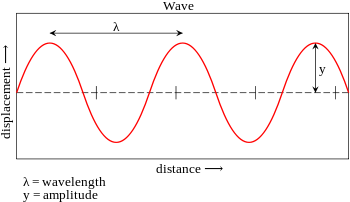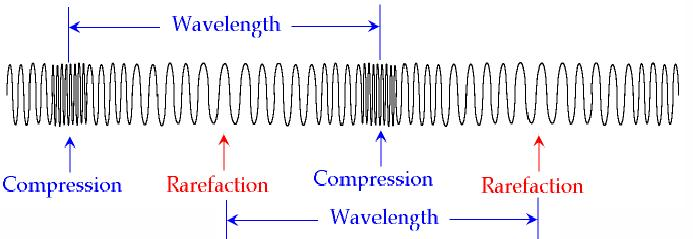Sound and Communication

Sound is a result of vibration. The vibration is produced by a source, travels in the medium as a wave and is ultimately sensed through the ear - drum.

Representative of Wave

A wave is represented in terms of its wavelength, amplitude, frequency and time period.Amplitude

The maximum height of the wave.

Wavelength

The distance between adjacent troughs or adjacent crests, measured in unit of length such as meters and expressed by symbol λ (lambda). For longitudinal wave, it will be distance between two successive rarefactions or compressions.

Time Period

This defines the time it takes for one complete wave to pass a given point, measured in seconds (s).

Frequency

The number of complete waves that pass a point in one second, measured in Hertz (Hz).

Speed or velocity

Wave speed is defined as the distance travelled by a wave disturbance in one second and is measured in meters/second (ms-1). Speed is scalar quantity while velocity is a vector quantity.

Period is inversely related to the frequency. This means if the frequency is high, the period will be low.

T = 1/n

Velocity = frequency × wavelength

V = n × λ

The waves that produce a sense of sound for living beings are called sound waves or audible waves. Only those waves that have frequencies lying in the range of 16 Hz to 20,000 Hz are audible to human beings.

Sound waves with frequencies below 16 Hz are called infrasonic waves and those above 20 kHz are ultrasonic waves.

Movement of Sound in Air

Sound waves travel in fluids and solids as longitudinal waves. A longitudinal wave is a wave in which vibration or the displacement takes place in the direction of the propagation of the wave. Sound moves due to difference in pressure. If a sound is produced in air, it compresses the adjacent molecules. Due to the compression, the air pressure increases.

This causes these compressed molecules to move in the direction of the pressure that is the direction of the wave. But displacement of the molecules causes fall in pressure in the place they left. If the wave is continuing then another rush of molecules comes in, fills the empty or rarified space. This process is repeated and the disturbance propagates. Thus a chain of compressions and rarefactions is generated due to sound. They travel and transport energy. If there is no medium, then produced sound will not be able to push any medium-molecules and sound will not move.

Different Types of Waves

The waves can be of different types. These may be mechanical or electromagnetic. Mechanical wave is a term used for those waves that require a medium for travelling. Its speed is dependent on the properties of the medium such as inertial and elastic properties. In other words the speed of the wave will depend on how easy or difficult is it to displace the particles of the medium (that is to say on their inertia) and on how those particles regain their original positions which is the elasticity.

An electromagnetic wave results from acceleration of charge. It doesn’t require a medium to travel. It can travel through vacuum such as light do waves which travel from stars through empty space to reach us. The electromagnetic wave has electric and magnetic fields associated with it. The 2 fields, electric and magnetic, are perpendicular to each other and also to the direction of propagation.

The Sound wave is a mechanical wave but light waves, infra red rays, X-rays, microwaves, Radio waves etc. are electromagnetic Gamma rays are alos em waves and result from radioactive decay of nuclei of atoms. Compared to sound waves, the em are much more energetic. They travel at the velocity of light that is about 3 lac kms per second in vacuum. In comparison, the sound waves travel very slowly. In air, it travels at 330 m s–1.

Mechanical wave can be either transverse or longitudinal while the electromagnetic wave is only transverse. The transverse wave is one in which the motion of wave and of the particles are perpendicular to each other. In a longitudinal wave, the motions are in the same direction. The sound wave can be of 2 types: Transverse and longitudinal.

In a longitudinal wave, the displacement of the particles and propagation of the wave are in the same direction. Just like the distance between two successive crests or troughs is a measure of wavelength for transverse waves, the distance between two successive compressions or rarefactions is termed wavelength of the longitudinal wave.While transverse waves form only in fluids (air and liquid), the longitudinal waves can form in all the three media viz. solid, liquid and gas.

Nature, Measure & Quality of Sound

Sound level is measured in units of decibel (dB). Here deci means one-tenth and bel is the level of sound. The term Bel is after the name of inventor of telephone Alexander Graham Bel.

Two power levels P1 and P2 are known to differ by n decibel if

n = 10 log10 P2/P1

Here P2 is the sound which is measured while P1 is a reference. Normally, the reference is a sound which is just audible. For average human ears, the whisper is about 30 decibel. The normal conversation is about 65 decibels while a jet plane taking off makes a noise of about 150 decibel. Beyond 85 decibels, sound is damaging and can lead to temporary loss of hearing. Prolonged exposure to noise can cause permanent hearing loss.

Voice is not just one frequency. It is a mix of many frequencies, some of which are multiples (called harmonics) of the same frequency called fundamental note for the person.